Next: Document representations and measures Up: irbook Previous: References and further reading   Contents   Index

# Vector space classification

The document representation in Naive Bayes is a sequence of terms or a binary vector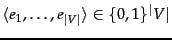. In this chapter we adopt a different representation for text classification, the vector space model, developed in Chapter 6 . It represents each document as a vector with one real-valued component, usually a tf-idf weight, for each term. Thus, the document space, the domain of the classification function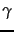, is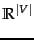. This chapter introduces a number of classification methods that operate on real-valued vectors.

The basic hypothesis in using the vector space model for classification is the contiguity hypothesis .

Contiguity hypothesis. Documents in the same class form a contiguous region and regions of different classes do not overlap.
There are many classification tasks, in particular the type of text classification that we encountered in Chapter 13 , where classes can be distinguished by word patterns. For example, documents in the class China tend to have high values on dimensions like Chinese, Beijing, and Mao whereas documents in the class UK tend to have high values for London, British and Queen. Documents of the two classes therefore form distinct contiguous regions as shown in Figure 14.1 and we can draw boundaries that separate them and classify new documents. How exactly this is done is the topic of this chapter.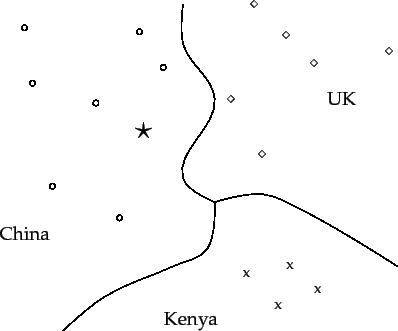Whether or not a set of documents is mapped into a contiguous region depends on the particular choices we make for the document representation: type of weighting, stop list etc. To see that the document representation is crucial, consider the two classes written by a group vs. written by a single person. Frequent occurrence of the first person pronoun I is evidence for the single-person class. But that information is likely deleted from the document representation if we use a stop list. If the document representation chosen is unfavorable, the contiguity hypothesis will not hold and successful vector space classification is not possible.

The same considerations that led us to prefer weighted representations, in particular length-normalized tf-idf representations, in Chapters 6 7 also apply here. For example, a term with 5 occurrences in a document should get a higher weight than a term with one occurrence, but a weight 5 times larger would give too much emphasis to the term. Unweighted and unnormalized counts should not be used in vector space classification.

We introduce two vector space classification methods in this chapter, Rocchio and kNN. Rocchio classification (Section 14.2 ) divides the vector space into regions centered on centroids or prototypes , one for each class, computed as the center of mass of all documents in the class. Rocchio classification is simple and efficient, but inaccurate if classes are not approximately spheres with similar radii.

kNN or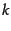nearest neighbor classification (Section 14.3 ) assigns the majority class of thenearest neighbors to a test document. kNN requires no explicit training and can use the unprocessed training set directly in classification. It is less efficient than other classification methods in classifying documents. If the training set is large, then kNN can handle non-spherical and other complex classes better than Rocchio.

A large number of text classifiers can be viewed as linear classifiers - classifiers that classify based on a simple linear combination of the features (Section 14.4 ). Such classifiers partition the space of features into regions separated by linear decision hyperplanes , in a manner to be detailed below. Because of the bias-variance tradeoff (Section 14.6 ) more complex nonlinear models are not systematically better than linear models. Nonlinear models have more parameters to fit on a limited amount of training data and are more likely to make mistakes for small and noisy data sets.

When applying two-class classifiers to problems with more than two classes, there are one-of tasks - a document must be assigned to exactly one of several mutually exclusive classes - and any-of tasks - a document can be assigned to any number of classes as we will explain in Section 14.5 . Two-class classifiers solve any-of problems and can be combined to solve one-of problems.

SubsectionsNext: Document representations and measures Up: irbook Previous: References and further reading   Contents   Index